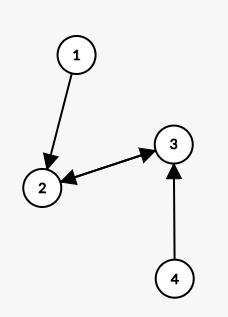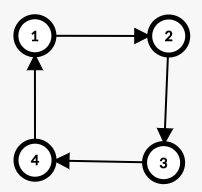Code

Code

Code

## DCode

## E

$$\left[\begin{matrix} 0 & 0 & 0 &0&0\\ 2^0 & 2^1 & 2^2&2^3&\ldots\\ 0 & 0&0&\ldots&\ldots\\ 2^2&2^3&\ldots&\ldots&\ldots\\ 0&\ldots&\ldots&\ldots&\ldots \end{matrix}\right]$$

Code

Code

## G

\begin{aligned} \omega &= \epsilon+\zeta\\ &=\epsilon+k-\alpha-\beta+\epsilon\\ &=2\epsilon+k-\alpha-\beta \end{aligned}

Code

## I

$V_1-E_1+F_1 = C_1+1$

$V_2-E_2+F_2=C_2+1$$C'_1 = F_2-Q_2$

$C'_2 = F_1-Q_1$

$$C_1-C_2-F_1+F_2 = V_1-E_1-V_2+E_2$$

\begin{aligned} \text{ans} &= C_1+C'_1-C_2-C'_2 \\ &= C_1+F_2-Q_2-C_2-F_1+Q_1\\ &= V_1-E_1-V_2+E_2+Q_1-Q_2 \end{aligned}

$a_i+b_j \geq x,a_i+b_{j+1} \geq x$

Code

Last modification：August 28th, 2020 at 02:07 pm